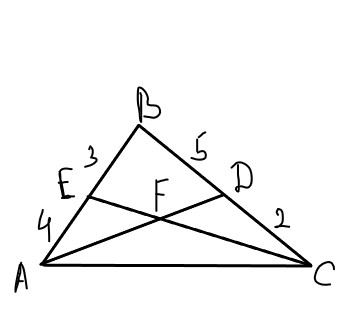Ishaan Booker

2022-07-16

In $\mathrm{△}ABC$, side BC is divided by D in a ratio of 5 to 2 and BA is divided by E in a ratio of 3 to 4 as shown in Figure. Find the ration in which F divides the cevians AD and CE i.e. find $EF:FC$ and $DF:FA$.yermarvg

Expert

Step 1
A general method to solving these kinds of things is through the Ratio Lemma, which is often applied in high school olympiad geometry. It states that if AD is a cevian in $\mathrm{△}ABC$, then $\frac{BD}{DC}=\frac{AB}{AC}\cdot \frac{\mathrm{sin}\mathrm{\angle }BAD}{\mathrm{sin}\mathrm{\angle }CAD}$. The proof of this is quite simple; just apply the sine law to triangles ABD and CAD.
Step 2
So for this problem, the ratios $\mathrm{sin}\mathrm{\angle }BAD:\mathrm{sin}\mathrm{\angle }CAD$ is clearly equal to $\mathrm{sin}\mathrm{\angle }EAF:\mathrm{sin}\mathrm{\angle }CAF$. So $EF:FC$ is equal to $\left(BD/DC\right)\left(AE/AB\right)=10/7$. $DF:FA$ is calculated similarly.
The Ratio Lemma can also be used to prove many basic facts in projective geometry; e.g. the invariance of the cross-ratio when projected through a point onto a line.Levi Rasmussen

Expert

Step 1
Use mass points. We assign B a mass of 4. So A has a mass of 3 and C has a mass of 10. E has a mass of 7 and D has a mass of 14.
Step 2
So we have $\frac{AF}{FD}=\frac{14}{3}$
then $\frac{EF}{FC}=\frac{10}{7}$

Do you have a similar question?